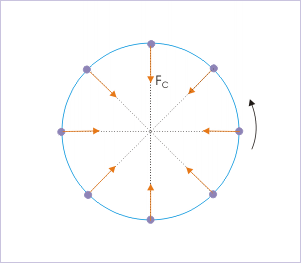# Dynamics of circular motion

 Page 1 / 3
Uniform circular motion requires a radial force that continuously change its direction, while keeping magnitude constant.

We have already studied the kinematics of circular motion . Specifically, we observed that a force, known as centripetal force, is required for a particle to execute circular motion.

Force is required because the particle executing circular motion needs to change direction continuously. In the case of uniform circular motion (UCM), speed of the particle is constant. The change in velocity is only in terms of change in direction. This needs a force in the radial direction to meet the requirement of the change in direction continuously.

The acceleration is given by : $\begin{array}{l}{a}_{r}=\frac{{v}^{2}}{r}={\omega }^{2}r\end{array}$

The centripetal force is given by :

$\begin{array}{l}{F}_{C}=m{a}_{r}=\frac{m{v}^{2}}{r}=m{\omega }^{2}r\end{array}$Uniform circular motion requires a radial force that continuously change its direction, while keeping magnitude constant.

## Centripetal force

The specific requirement of a continuously changing radial force is not easy to meet by mechanical arrangement. The requirement means that force should continuously change its direction along with particle. It is a tall order. Particularly, if we think of managing force by physically changing the mechanism that applies force. Fortunately, natural and many craftily thought out arrangements create situations, in which the force on the body changes direction with the change in the position of the particle - by the very act of motion. One such arrangement is solar system in which gravitational force on the planet is always radial.

Centripetal force is a name given to the force required for circular motion. The net component of external forces which meet this requirement is called centripetal force. In this sense, centripetal force is not a separately existing force. Rather, we should look at this force as component of the external forces on the body in radial direction.

## Direction of centripetal force and circular trajectory

There is a subtle point about circular motion with regard to the direction of force as applied on the particle in circular motion. If we apply force on a particle at rest, then it moves in the direction of applied force and not perpendicular to it. In circular motion, the situation is different. We apply force (centripetal) to a particle, which is already moving in a direction perpendicular to the force. As such, the resulting motion from the interaction of motion with external force is not in radial direction, but in tangential direction.

In accordance with Newton's second law of motion, the particle accelerates along the direction of centripetal force i.e. towards center. As such, the particle actually transverses a downward displacement (Δy) with centripetal acceleration; but in the same time, the particle moves sideways (Δx) with constant speed, as the component of centripetal force in the perpendicular direction is zero.

It may sound bizzare, but the fact is that the particle is continuously falling towards the center in the direction of centripetal force and at the same is able to maintain its linear distance from the center, owing to constant side way motion.

pls explain what is dimension of 1in length and -1 in time ,what's is there difference between them
what are scalars
show that 1w= 10^7ergs^-1
what's lamin's theorems and it's mathematics representative
if the wavelength is double,what is the frequency of the wave
What are the system of units
A stone propelled from a catapult with a speed of 50ms-1 attains a height of 100m. Calculate the time of flight, calculate the angle of projection, calculate the range attained
58asagravitasnal firce
Amar
water boil at 100 and why
what is upper limit of speed
what temperature is 0 k
Riya
0k is the lower limit of the themordynamic scale which is equalt to -273 In celcius scale
Mustapha
How MKS system is the subset of SI system?
which colour has the shortest wavelength in the white light spectrum
if x=a-b, a=5.8cm b=3.22 cm find percentage error in x
x=5.8-3.22 x=2.58
what is the definition of resolution of forcesBy OpenStaxBy Kevin AmaratungaByByBy Brianna BeckBy OpenStaxBy OpenStaxBy Vanessa SoledadBy OpenStaxBy Carly Allen